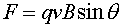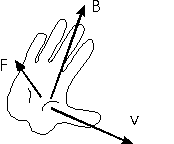The right-hand rule Remember the magnitude of the force felt by a charge q moving with velocity v through a magnetic field B is:As stated previously, the direction of the force is perpendicular to both v and B. Of course, if both v and B are pointed in the plane of the screen, F could be either into or out of the screen to be perpendicular to both vectors. One uses the right-hand rule to determine the direction of F.To find the direction of F use your right hand and: Point your thumb into the direction of v. Point your fingers into the direction of B. The direction of the force will be out of your palm. Your fingers will curl into the direction of the force. If the charge is negative, one must remember the direction of the force will be opposite. To calculate the force on a segment of wire, use the direction of the current I instead of v. If you had used your left hand the force would have been directed oppositely. It seems as if this violates fundamental American principles of equality. However, we will learn that the direction of B is also determined through a right-hand rule, and the application of two right-hand rules to get to something meaningful (the force) means that two left-hand rules would have given the same result. Thus the laws of electromagnetism do not favor right-handed vs. left-handed people, they only favor consistency. If a physical result depended on the right-handed rule that would constitute violation of parity. This indeed occurs in weak decays which will be studied at the end of this course. In weak decays, if one considers a nucleus where the charge rotates as your fingers wrap around your right hand, the emitted electrons all go along the direction of your thumb. Curiously enough, if one did the same experiment with antimatter, one would have to use one's left hand to find the direction of the emitted antielectrons.